﻿ Improving the Statistical Capacity Index: A Statistical ApproachPublications are Open
Access in this journal
Article Versions
Export Article
• Normal Style
• MLA Style
• APA Style
• Chicago Style
Research Article
Open Access Peer-reviewed

### Improving the Statistical Capacity Index: A Statistical Approach

Dharmaratne MA , Attygalle MDT
American Journal of Applied Mathematics and Statistics. 2018, 6(3), 80-95. DOI: 10.12691/ajams-6-3-1
Published online: May 22, 2018

### Abstract

Good quality, timely and accurate statistics lie at the heart of a country's effort to improve development effectiveness. As a response to the challenge of measuring the institutional capacity of a country in producing timely and accurate statistics, the World Bank developed its framework for the Statistical Capacity Index (SCI). Although the World Bank's framework is acknowledged for its simplistic approach, it has received extensive critique for the ad-hoc allocation of weights. This research attempts to find a solution to this criticism using a statistical methodology. Country information used by the World Bank to create the SCI for the year 2014 was considered. The data consisted information on 25 categorical variables out of which 16 were binary variables and 9 were ordinal variables. Nonlinear Principal Component Analysis (NLPCA) was conducted on the categorical data to reduce the observed variables to uncorrelated principal components. Consequently, the optimally scaled variables were used as input for factor analysis with principal component extraction. The results of the factor analysis were used to weight the new SCI. The dimension, availability and periodicity of economic and financial indicators explained most of the variance in the data set. The research proposes a simpler version of the new SCI with only 23 variables. In the proposed new index, the variables enrolment reporting to UNESCO, gender equality in education and primary completion indicators were the three variables receiving the largest weight. These three indicators measure the periodicity of reporting data on educational statistics to UNESCO; periodicity of observing the gross enrolment rate of girls to boys in primary and secondary education; and periodicity of observing the PCR indicator which is the number of children reaching the last year of primary school net of repeaters respectively. This research represents the first attempt to create a SCI using multivariate statistical techniques and especially index construction with NLPCA. The research concluded with a comparison of the proposed new index and the index created by the World Bank, which justified that the proposed index be used as a solution for the arbitrary allocation of weights in creating the SCI.

### 1. Introduction

Statistical Capacity is defined as "a nation's ability to collect, analyze, and disseminate high-quality data about its population and economy" 17. The World Bank has been actively involved in strengthening the statistical capacity of developing countries particularly through investment in national statistical systems. This has paved way for the greater need to measure the progress and results of these projects. As a response to this need, the World Bank has been rating over 140 developing countries for their statistical capacity.

The Statistical Capacity Indicator (SCI) was developed by the World Bank to assess the national statistical capacity of over 140 developing countries. The SCI is accessible through the World Bank website and it is been updated annually since its inception in 2004. The diagnostic framework of the SCI uses metadata information available for most countries to monitor progress in statistical capacity building over time. The framework adopted by the World Bank has three main dimensions namely, statistical methodology, source data, periodicity and timeliness. The countries are scored against specific criteria using information on 25 sub indicators available from the World Bank, UNESCO, UN, WHO, and IMF 16 (See Appendix A).

The multi-dimensional approach followed by the World Bank in developing the SCI is based on the intuition that producing and disseminating quality statistics require a certain level of capacity in all of the three dimensions. An imbalance in any one of the three dimensions would indicate a weakness in some aspects of the statistical process. Hence, this type of composite measure would shed light on data quality and on areas that the countries need to focus on improving to produce and disseminate reliable and timely statistics.

According to 5, the primary advantage of the methodology adopted by the World Bank is that metadata from existing databases of international organizations can be used in the compilation of the indicators. Hence, the countries will not have the additional burden of data collection, reporting and country visits. In addition, through this approach the consistency of information across countries and time will be preserved.

However, there are some apparent issues in the statistical capacity indicator developed by the World Bank. Reference 15 report has highlighted some potential issues of the current SCI. The first problem is the choice of the indicators used to measure statistical capacity. The second problem is that the SCI mainly capture the availability of statistical outputs rather than the outcomes and impacts of improved statistical capacity. Furthermore, according to Reference 13, only six items out of the twenty-five indicators encompassing the SCI actually relate to statistical capacity aspects and "as many as 19 items (accounting for around 77% of the overall score) relate to statistical activities and outputs" [ 4, p.158].

Moreover, both 15 and 13 discuss the limitations of the SCI in the context of the choice of the weights used and the method of aggregation. Reference 13 believes that some of the weaknesses in the current SCI can be avoided by improved method of aggregation by minimizing the weights allocated to statistical activities and outputs.

In addition, the possibility of using statistical methodologies and techniques of index construction have not been explored in the development of the SCI. Literature reveals that multivariate techniques can be used for index creation and thus may be used to derive more appropriate and sensible weights for the twenty-five indicators of the current index. Therefore, the key objective of this study is to explore the possibility of using multivariate techniques and improving the statistical capacity index.

Several different weighting techniques exist derived on statistical models or on participatory methods. Statistical methods to index construction mainly base on weights derived using techniques such as factor analysis, data envelopment analysis and unobserved components models 14. Reference 1 discusses the use of multivariate methods applicable in index construction where a wealth index is constructed using principal component analysis. Similarly, 7 has developed a socioeconomic index to differentiate disadvantaged areas from the more privileged areas using Principal component analysis (PCA) and Factor analysis (FA). Various other studies too have adopted similar methodologies of index construction and have extensively reviewed the use of PCA in constructing socio-economic status indices, their validity and limitations. Furthermore, 3 explored the possibility of using Principal component analysis in deriving the Human development index (HDI). However, the PCA method yielded weights that are nearly identical to those used in the HDI and hence the simplistic approach in the existing HDI was preferred.

Nevertheless, Principal component analysis and Factor analysis are widely used data reduction techniques for continuous data. Hence, PCA or FA is not suitable for categorical data as treating categorical variables as continuous variables are not meaningful. The handbook on constructing composite indicators 14 suggests the use of Correspondence Analysis (CA) in constructing composite indicators when there are categorical variables. To analyze the relationship among several categorical variables an extension of CA, Multiple correspondence analysis (MCA) can be used.

Reference 2, used Multiple correspondence analysis to develop a multi-dimensional poverty indicator. Results obtained from MCA can be interpreted similar to a PCA solution. In deriving the poverty indicator 2 has only retained two factorial axes as the two axes together explain sufficient amount of inertia (variance) in the data set. The weights for the index are derived using the factorial scores of the variables in the first two axes. In fact, MCA is the oldest application of PCA to categorical variables. However, 12 raise concerns over the interpretability of MCA solutions as they are expressed in terms of combinations of categories for each of the individual variables. This will complicate the interpretability of the MCA results, because MCA yields factorial scores for each level of the categorical variables. Hence, indices based on MCA will have weights for each level of the categorical variable. Thus, these types of indices will have little practical use over the long term.

Nonlinear Principal Component Analysis (NLPCA) also known as Categorical Principal Component Analysis (CATPCA) is an alternative to PCA, that is useful for analyzing multivariate data with different measurement levels. Similar to PCA Nonlinear Principal Component Analysis is also a data reduction technique, which in addition can reveal nonlinear relationships among the variables. The basic methodology behind NLPCA is the transformation of the categories of variables with nominal and ordinal analysis level to numeric values using a process called optimal scaling. Hence, these transformations will be optimal to the model being fitted 9.

Many researchers have not explored the use of NLPCA in index construction as it is a relatively new approach. However, NLPCA is able to overcome most of the limitations of the traditional PCA and MCA methods. NLPCA produces a similar output to that of PCA with eigenvalues, component loadings and communalities 9. Reference 12, also argue the use of NLPCA over other methods stating that the interpretability of the NLPCA solutions are much enhanced compared to MCA solutions as NLPCA produces components that are combinations of the variables instead of categories. Hence, weights for the index can be derived from the NLPCA solution in a similar fashion to that of weights derived using PCA or FA.

The study involves creating a new statistical capacity index by obtaining weights for the index using statistical methodologies. Nonlinear principal component analysis is used to optimally transform the categorical variables into interval scale variables. To enhance the interpretability of the component loadings a standard factor analysis is performed with the optimally scaled variables. The rotated component loadings of factor analysis will be then used to estimate the weights for the composite indicator.

Section1 gives an introduction to the study. The second section gives the theories and methods used throughout this study. The third section consists of the results of the statistical analysis. The final section contains the conclusion on the findings of the analysis conducted in this research.

### 2. Methodology

2.1. Nonlinear Principal Component Analysis

Nonlinear Principal Component analysis is the nonlinear counter part of standard principal component analysis, and similar to PCA it reduces the observed variables to uncorrelated principal components. In NLPCA, every category is converted to a numeric value with respect to the variable's analysis level using optimal scaling and the standard PCA is then performed on the transformed variables.

2.1.1. Nonlinear Principal Component Analysis Using Aspects

In Mair and de Leeuw (2010) cited in 4 the aspect package in R is described. The theory from de Leeuw (1988) cited in 4, is implemented and it is another way to arrive at NLPCA.

Consider the multivariate dataset with m variables and the correlation matrix R(Y). The aspect ϕ describes the criterion to optimize and Y = (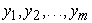) with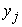as the vector of category scores to be computed. The optimization problem can be formulated simply as in equation (1),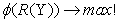(1)

For nonlinear principal component analysis Eigenvalue aspects ("aspectEigen") are used. The basic definition of an eigenvalue aspect is to maximize the largest eigenvalue λ of R. As a multidimensional generalization, can look at the sum of the first p eigenvalues as shown in equation (2),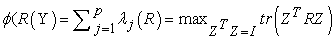(2)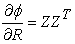Where Z is the m×p matrix of the first p eigenvectors. This generalization is used in nonlinear PCA. The aspect is convex because it is the point wise maximum of a family of linear functions. Hence, majorization of eigenvalue aspect over transformations is guaranteed to converge.

Source: 10

Conducting a NLPCA is a dynamic process in which the researcher plays an active role. A series of steps need to be followed while evaluating analysis results at each step and revising analysis options as required.

2.1.2. Determining the Analysis Level of the Variables

The researcher needs to specify a nominal, ordinal, or numeric analysis level for each variable in the data set. The analysis level of the variable determines the amount of freedom allowed in transforming category values to category quantifications.

2.1.3. Determining the Appropriate Number of Components

In a nonlinear PCA, the number of components will affect the analysis results, in NLPCA eigenvalues of the first P components are maximized. Two of the most frequently used methods are described below.

• Kaiser's eigenvalue greater than one rule

According to the eigenvalue greater than one rule, only the factors with eigenvalues greater than one will be retained for interpretation 8.

• Cattell's Scree test

In this method, the plot of eigenvalues in descending order is examined to identify the point at which a significant break takes place. In other words, the point at which a significant "elbow" is seen indicates the suitable number of components to be retained in the solution.

2.1.4. Rotation of NLPCA Solution

Once a NLPCA is conducted, it will be required to interpret the results of the solution by inspecting the component loadings. However, unrotated solutions are often difficult to interpret. Reference 11 have used the transformed variables as input for a factor analysis with principal component factor extraction and varimax rotation (as the unrotated results of this is identical to the NLPCA solution).

2.2. Factor Analysis

Factor analysis attempts to identify underlying yet unobservable random quantities called factors that explain the pattern of correlations within a set observed variables.

Let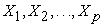denote the observed responses. Let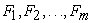denote the latent factors where m<p. The factors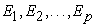each of which affecting only one response variable, are called Specific factors. Then the factor analysis model with m common factors can be written as in equation (3) in matrix notation; where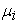is the mean of variable i.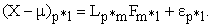(3)

The unobservable random vectors F and ε satisfy;

• F and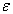are independent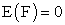,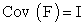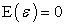,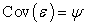, where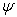is a diagonal matrix

Source: 6

2.2.1. Measures of Appropriateness of Factor Analysis

Prior to the extraction of the factors, the suitability of the data for factor analysis should be assessed. The two commonly used tests include Kaiser-Meyer-Olkin (KMO) Measure of Sampling Adequacy and Bartlett's Test of Sphericity.

If the test is not significant, do not use factor analysis as the factors will not load together well. In addition, weights for constructing an index can only be estimated if there are significant correlations among the indicators.

Once the suitability of the data for factor analysis is established, principal component factor analysis with Varimax rotation is performed on the data set.

2.3. Weighting System and Index Construction

Once the factor analysis of optimally scaled variables with varimax rotation is performed the factors from the resulting solution will be used to calculate the composite indicator of statistical capacity. The following steps were followed to construct the index using a novel approach to index construction as described by Nicoletti et al. (2000) cited in 14.

• Calculate the squared factor loadings for each variable under each of the extracted factors. These correspond to the weights of each variable.

• Group the individual indicators with the highest factors loadings into intermediate composite indicators.

• The intermediate composites are aggregated by assigning a weight to each one of them equal to the proportion of the variance explained in the data set.

### 3. Statistical Analysis

Nonlinear principal component analysis is used to optimally transform the categorical variables into interval scale and identify any nonlinear relationships among the variables. Nonlinear principal component analysis for many dimensions is performed to find the most appropriate number of dimensions. Then the optimally scaled variables from the NLPCA are used as the input for factor analysis with principal component extraction method. The results of the factor analysis are used to derive weights for the final composite index.

The data set used in the analytical process consists of data on 25 variables for 145 developing countries. All the variables are categorical variables with 16 binary variables and 9 ordinal categorical variables (See Appendix A).

3.1. Analysis Level of the Variables

The first step in performing a NLPCA is the specification of the analysis level for each variable in the data set. Since there is an inherent ordinal nature to the data set, all the variables will be analyzed at the ordinal level. The category order in the quantifications is maintained both on theoretical grounds and for the ease of interpretation.

3.2. Number of Components

In determining the number of components, results from different number of components need to be compared. Kaiser's eigenvalue greater than one criterion suggests a 7-dimensional solution (the eigenvalues only vary slightly). However, Catell's scree test suggests a 5-dimensional solution.

Since the number of components to be retained suggested by the two criteria used was different, a third criterion: interpretability of the components was considered. Since the interpretation of the sixth and seventh components in the 7-dimensional solution are unclear (see Appendix B2), the number of components selected for the final NLPCA solution was five.

3.3. Nonlinear Principal Component Analysis 5-dimensional solution

It can be seen that there are seven eigenvalues greater than one. The proportion of variance explained by the seven components together is around 64%. However, based on the Scree test and interpretability of factors, a 5-dimensional solution was selected. According to Table 1, it is evident that the first five components together explain approximately around 55% of the variation in the data. This amount is significantly high and is sufficient for further analysis of the data.

From Table 2 it is clear that except for the variable National accounts base year (A), the proportion of variance explained by the common factors for the remaining twenty-four variables is approximately greater than 0.3, which is denoted by the communality values.

Next, the significance of the component loadings need to be assessed. According to Stevens (1992), a cut-off of 0.4 on the factor loading irrespective of the sample size will be adequate for interpretive purposes.

Careful examination of Table 2 would reveal that while most of the variables are highly loaded on one of the five factors, there are few variables, which are moderately loaded on several factors. Hence factor rotation is performed as this made the interpretation of the factors quite difficult. Since there are no rotation options available in a usual NLPCA, the transformed variables will be used as inputs to a Factor analysis with Principal component factor extraction and varimax rotation.

3.4. Testing the Appropriateness of a Factor Analysis

Before performing a factor analysis, the appropriateness of the factor analysis was tested for the transformed variables. The Kaiser-Meyer-Olkin (KMO) measure of sample adequacy test produced an overall KMO statistic value of 0.77. Since the overall KMO value is greater than 0.6, the value is higher enough to proceed with the factor analysis. Also, Bartlett’s Test of Sphericity was performed to test the strength of the relationship among the variables. The Bartlett’s Test of Sphericity tests the null hypothesis "the correlation matrix is an identity matrix". The results of the analysis showed a p value approximately equal to zero. Hence, the test is significant and can proceed with the factor analysis.

3.5. Results of the Factor Analysis

Table 3 presents the results of the factor analysis with varimax rotation. It can be seen that the two variables National accounts base year (A) and Periodicity of agricultural census (L) have factor loadings less than 0.400 and therefore these two variables have not loaded for any of the five factors. Thus, these two variables were not included in any one of the five factors.

The first factor alone explains about 29.4% of the variation in the data out of the five factors extracted (Refer Appendix E1). The first factor is heavily loaded on the variables; IMF’s Special Data Dissemination Standard (I), Industrial production index (E) and Periodicity of income poverty indicator (W). IMF’s Special Data Dissemination Standard looks at the availability of timely and comprehensive economic and financial data in IMF's Bulletin Board. Hence, the first factor mostly looks at the availability and periodicity of economic and financial indicators. The first factor is also significantly loaded on the variables, Periodicity of poverty related surveys (M), Government finance accounting concept (G), Import/export prices (F) and moderately loaded on the variable Consumer Price Index base year (D), which are also indicators related to either economic or financial data.

The second factor is positively loaded on the variables Periodicity of child malnutrition indicator (R), Periodicity of health-related surveys (N) and HIV/AIDS indicator (V) while it is negatively loaded on the variables Vaccine reporting to WHO (J) and Completeness of vital registration system (O). The variable Completeness of vital registration system measures the availability of complete registries of birth and death statistics. It is evident that the second factor relates to health indicators and mainly captures the availability and periodicity of health-related indicators. However, it is important to note the negative signs of the factor loadings in the two variables J and O. This means that countries who perform better on the variables Periodicity of child malnutrition indicator, Periodicity of health related surveys and HIV/AIDS indicator will perform poorly in the variables Vaccine reporting to WHO and Completeness of vital registration system and vice versa.

The third and fourth factors roughly explain around 18% of the variation of the first five factors each. The third factor is highly loaded on the variables, Periodicity of child mortality indicator (S), Periodicity of Immunization indicator (Q), Access to water indicator (P) and quite significantly with Periodicity of maternal health indicator (X). It is evident that except for the Access to water indicator, the other three indicators measure the periodicity of child/mother health indicators.

The fourth factor has only three variables, which are all heavily loaded on the fourth factor. The interpretation of the fourth factor is quite straightforward with all the three indicators attempting to measure the quality of education provided in a country. The Primary completion indicator (Y) mainly captures the number of children reaching the last year of primary school. The periodicity of reporting data on education to UNESCO Institute of Statistics by a country is measured by the variable, Enrolment reporting to UNESCO (H). Similarly, Periodicity of gender equality in education indicator (T) provides an overview on the equality of educational opportunities provided by a country.

The final factor, which is the fifth factor that accounts for the least amount of variance among the extracted factors, can be interpreted similar to the first factor. This factor is highly loaded on the variable Periodicity of GDP growth indicator (U) while being almost equally loaded on the three remaining variables. Although the four variables capture quite different aspects of economic data, overall the fifth factor too can be interpreted as a factor related to economic or financial indicators.

3.5.1. Adequacy of the Fitted Model

Once a factor analysis is carried out, the residual correlation matrix was inspected to ensure the adequacy of the fitted model. In the residual correlation matrix with uniqueness on the diagonal, it was seen that while most of the residual correlations are of order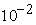, there were some residual correlation values between 0.1 and 0.3. However, given the nature of these 25 variables and the fact that only 55% of the variance in the data set is being accounted for, the residual correlations are small enough to conclude that the fitted factor model is adequate.

3.5. Constructing the Statistical Capacity Indicator Using Multivariate Methods

Factor analysis can be used to group together individual indicators that are correlated to form an index, which accounts for as much as possible information common to the individual indicators. Therefore, the results of the factor analysis in the previous section were used to construct the index for measuring statistical capacity of developing countries.

3.5.1. Weighting and Aggregation

The new index for measuring the statistical capacity of developing countries will be calculated using the approach explained in 14.

The matrix of factor loadings after rotation in Table 3 was used to calculate the weights for each of the 23 variables. Given that the square of the factor loadings represents the proportion of the total unit variance of the indicator explained by the factor, the weights for each of the indicators will be the normalized squared factor loadings.

In Table 5 the normalized squared factor loadings of the 5-dimensional factor solution with varimax rotation is depicted. The normalized squared factor loading for each variable is its corresponding squared factor loading divided by the variance explained by the respective factor. The variance explained by each of the factors of the 5-dimrnsional factor solution with varimax rotation can be seen in Appendix B1.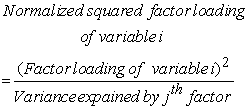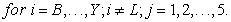For example, the normalized squared factor loading of variable I under factor 1 would be;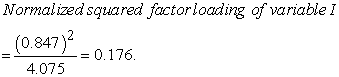In the above example: 0.176 is the portion of the variance of the first factor explained by the variable, IMF’s Special Data Dissemination Standard (I).

In the above table the highlighted values correspond to the variables with high factor loadings (>0.4). These are the corresponding weights for each of the 23 variables.

The last step in the construction of an index is the aggregation of the individual variables into a single composite index. The approach used by  was used to aggregate the variables and form the index. Based on this approach there are five intermediate composite indicators (Table 4) with weights for each variable given in Table 5.

In the table in Appendix E1 it can be seen that the variance explained by each of the factors is different. The five-factor model with varimax rotation explains about 55.4% of the total variation in the data with 16.3% by the 1st factor, 11.4% by the 2nd factor, 10% by the 3rd factor, 9.7% by the 4th factor and 8% by the 5th factor. Therefore, the importance of the factors measuring the overall statistical capacity is not the same. Hence, the five intermediate composite indicators are aggregated by assigning a weight to each one of them equal to the proportion of the variance explained in the data set. The proportion of variance explained is calculated as follows: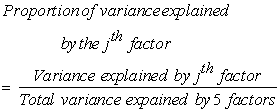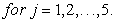For example;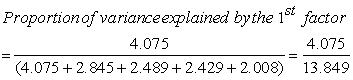The five intermediate composites are aggregated by assigning a weight to each one of them equal to the proportion of the explained variance in the data set. Therefore, each variable weight is multiplied by the corresponding factor weight for the final index calculation.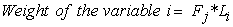(4)Where,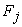is the Factor weight of jth factor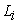is the Normalized squared factor loading of variable i.

Therefore, the new SCI can be calculated as follows;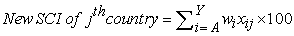(5)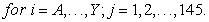where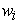= weight of the ith variable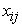= score of the ith variable for jth country

For index values of the countries according to the New statistical capacity index, refer Appendix C1.

3.5. Proposed Statistical Capacity Indicator

It can be seen that the new statistical capacity index constructed using the results of the factor analysis of optimally scaled variables yielded weights for each variable. That is there are weights for each of the 23 variables separately. However, in reality when the statistical capacity indicator is to be calculated annually for over 140 developing countries, having different weights for 23 variables will be cumbersome. In addition, the weights derived for the variables are specific to that data set only.

Therefore, a more general approach for calculating the index in future is proposed. The weights for the new index are calculated based on the proportion of the variance explained by each factor. Thus;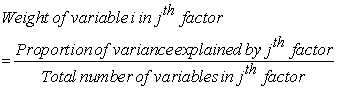Therefore, the Proposed statistical capacity indicator can be calculated as follows;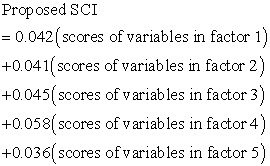For index values of the countries according to the Proposed statistical capacity index Appendix C1.

3.6. Comparison of Proposed SCI and New SCI

A comparison of the New SCI developed using statistical methodologies and the Proposed SCI was made, by drawing the scatter plot of the statistical capacity indicator values of the two indices. It was confirmed that the two indices were almost the same by the high R2 value of 0.988 indicating a good fit in the data.

Therefore, it can be concluded that the Proposed SCI is capable of producing statistical capacity indicator values consistent with the New SCI developed using statistical methods. Hence, the weights of the Proposed SCI can be used in future to measure the statistical capacities of developing countries.

3.7. Comparison of Proposed SCI and SCI by the WB

As mentioned in the introduction, one of the main motivational factors of this study was to obtain weights for the twenty-five variables using statistical methodologies. This is due to the fact that, one of the main criticisms against the statistical capacity index developed by the World Bank is the arbitrary allocation of weights that lack theoretical validity. Table 9 below provides a comparison of the variable weights according to the current index and the proposed index.

Comparison of the results in Table 9 reveals that based on the five factors extracted from the factor analysis during the advanced analysis, the proposed index is composed of only 23 variables. In the current SCI the five variables in the second dimension, source data receive the largest weight of 6.672 with the remaining fifteen variables receiving each an equal weight of 3.332. According to the proposed index the variables Enrolment reporting to UNESCO, Periodicity of gender equality in education indicator and Primary completion indicator are the three variables receiving the largest weight of 5.849. However, in the current index these three variables are only weighted by 3.332 units. Therefore, according to the proposed index, the most important aspect of determining the statistical capacity of a country is the periodicity of educational indicators that capture the quality of education provided and the equality of educational opportunities in a country.

The variables, Access to water indicator, Periodicity of Immunization indicator, Periodicity of child mortality indicator and Periodicity of maternal health indicator are weighted approximately by 4.5 units in the proposed index. In the current index, these four indicators too receive an equal weight of 3.332 each. Hence, the second most important aspect in measuring statistical capacity according to the proposed index is mostly based on the periodicity of child/mother health indicators.

It is also noteworthy that the five variables receiving the highest weight according to the current SCI are receiving relatively low weights of around 4.2 to 3.6 units in the proposed SCI. However, the highest weight received by a variable in the proposed index (5.849) is less than that of the current index (6.672). The variable Periodicity of agricultural census, which is highly weighted in the current index, does not contribute in the formation of the proposed index. According to the results of the preliminary analysis, 63% of the countries have conducted an agricultural census during the past 10 years. Similarly, the other variable that was dropped from the proposed index, National accounts base year also has about 64% of the countries scoring the maximum possible mark.

Comparing the weights in the proposed index with the results of the descriptive analysis further revealed that two of the variables receiving the highest weights Periodicity of gender equality in education indicator and Primary completion indicator have less than 40% of the countries scoring the maximum possible value. Similarly, the variables Import/export prices, IMF’s Special Data Dissemination Standard, Industrial production index, Government finance accounting concept and Periodicity of income poverty indicator that are relatively important contributors to the proposed index (with weights of 4.205) are also having less than 50% countries adhering to the ideal standards.

3.8. Validation of Proposed SCI

The index values and ranks according to the proposed index for the year 2013 are compared with the index values of SCI by WB for 2013.

Results of the index values and ranks can be seen in Appendix C2 (arranged in ascending order of ranks according to the proposed index). It can be seen that the ranking of countries according to the proposed SCI for the year 2013 seem to be reasonable. Most of the countries among the top 10 are either upper middle-income or lower middle-income countries. Whereas the countries in the bottom 10 ranks are mostly either low-income or lower middle-income countries.

Furthermore, it was pointed out earlier that statistical capacity building is a long-term process. Hence, ideally the SCI should not be volatile in the short term. Therefore, if the SCI adequately captures the statistical capacity of developing countries, there cannot be huge year on year variations in the index. From Appendix C2 it is evident that in comparing the SCI values for years 2013 and 2014 calculated based on the proposed approach, for most of the countries the index values for the two years are quite similar with differences between the indices for the two years amounting to less than 10 units for most countries. This further proves that the proposed SCI can be used for future calculation of statistical capacity of developing countries, where the index has built up on a sound statistical methodology

### 4. Conclusion

During the past couple of decades the world has witnessed a trend in international community working together to fill the gaps in the demand for official statistics. As a response to the challenge of measuring the institutional capacity of a country in producing timely and accurate statistics, the World Bank developed its framework for the statistical capacity index. Despite the fact that the SCI developed by the World Bank do not have the additional burden of data collection and reporting for a country, the index is criticized for its ad-hoc allocation of weights. The methodology followed by the World Bank in weighting and aggregating the twenty-five variables has received extensive critique.

This research was therefore based on estimating sensible weights for the variables and aggregating the variables to form a composite indicator for measuring the statistical capacity of developing countries. The focus of this study was to employ statistical methodologies of index construction as no previous attempts have been made to derive weights for the statistical capacity index using statistical methods.

A simpler Statistical Capacity Index is proposed taking into account only the proportion of variance explained by each of the factors of the factor analysis. Although the proposed SCI was not able to fully mitigate the shortcomings of the SCI created by the World Bank, this study was able to address the issue of arbitrary allocation of weights in aggregating the variables to form a composite indicator which has been a major criticism against the SCI created by the World Bank. The solution proposed by this research by employing multivariate statistical theories and methodologies successfully addresses these issues and make suggestions for an improved statistical capacity index with five meaningful dimensions. Finally, the creation of the proposed index maintains the simplicity in calculating while also justifying the weights used for its creation.

### Acknowledgements

I would like to extend my sincere gratitude to all the lecturers of the Department of Statistics, University of Colombo for supporting me in numerous ways during this study.

### Abbreviations

SCI: Statistical Capacity Index

NLPCA: Nonlinear Principal Component Analysis

PCA: Principal Component Analysis

WB: World Bank

FA: Factor Analysis

CA: Correspondence Analysis

MCA: Multiple Correspondence Analysis

### References

  Abeyasekera, S. (2006). Chapter 18: Multivariate methods for index construction. Household Surveys in Developing and Transition Countries: Design, Implementation and Analysis. Retrieved June 26, 2015, from http://unstats.un.org/unsd/hhsurveys/finalpublication/ ch18fin3.pdf. In article View Article  Asselin, L. M. (2009). Analysis of Multidimensional Poverty: Theory and Case Studies. (Vol. 7). New York: Springer Science+Business Media. In article View Article  Biswas, B., & Caliendo, F. (2002). A Multivariate Analysis of the Human Development Index. Economic Research Institute: Study Paper No. 244. Retrieved September 20, 2015, from http://digitalcommons.usu.edu/eri/244 In article View Article  de Leeuw, J. (2013). History of nonlinear principal component analysis. Retrieved November 2, 2015 from statistics.ucla.edu /.../History%20of%20Nonlinear%20Principal%20Comp. In article View Article  Fantom, N., & Watanabe, N. (2008). Improving the World Bank’s Database of Statistical Capacity. African Statistical Newsletter, 2(3), 21-22. Retrieved September 2015, from http://www.uneca.org/sites/default/files/PageAttachments/asn_june2008.pdf. In article View Article  Johnson, R. A., & Wichern, D. W. (2007). Applied Multivariate Statistical Analysis. (6th ed.). New Jersey: Pearson, Prentice Hall. In article  Krishnan, V. (2010). Constructing an Area-based Socioeconomic Index: A Principal Components Analysis Approach. Early Child Development Mapping Project Alberta: Canada. Retrieved September 18, 2015 from http://www.cup.ualberta.ca/wp-content/uploads/2013/04/SEICUPWebsite_10April13.pdf. In article View Article  Ledesma, R. D., & Valero-Mora, P. (2007). Determining the Number of Factors to Retain in EFA: an easy-to use computer program for carrying out Parallel Analysis. Practical Assessment, Research & Evaluation, 12(2). Retrieved October 30, 2015 form http://pareonline.net/pdf/v12n2.pdf. In article View Article  Linting, M., & van der Kooij, A. (2012). Nonlinear Principal Component Analysis With CATPCA: A Tutorial. Journal of Personality Assessment, 94(1), 12-25. In article View Article  PubMed  Mair, P., & de Leeuw, J. (2010). A General Framework for Multivariate Analysis with Optimal Scaling: The R Package aspect. Journal of Statistical Software, 32(9). Retrieved October 18, 2015 from http://www.jstatsoft.org/article/view/v032i09/v32i09.pdf. In article View Article  Manisera, M., van der Kooij, A. J., & Dusseldorp, E. (2010). Identifying the Component Structure of Satisfaction Scales by Nonlinear Principal Component Analysis. Quality Technology & Quantitative Management, 7(2), 97-115. Retrieved October, 2015. In article View Article  Merola, G., & Baulch, B. (2014). Using Sparse Categorical Principal Components to Estimate Asset Indices New Methods with an Application to Rural South East Asia. Paper presented at conference ABSRC Conference, September 24-26, 2014. Rome. Retrieved October 30, 2015 from http://veam.org/papers2014/43_Giovanni%20Merola-Baulch%20-%20USING%20SPARSE%20 CATEGORICAL% 20PRINCIPAL.pdf. In article View Article  Ngaruko, F. (2008). The World Bank’s Framework for Statistical Capacity Measurement: Strengths, Weaknesses, and Options for Improvement. The African Statistical Journal, 7, 149-169. Retrieved June 12, 2015. In article View Article  Organization for Economic Co-operation and Development (OECD). (2008). Handbook on Constructing Composite Indicators: Methodology And User Guide [online book]. Retrieved August 8, 2015, from http://www.oecd.org/std/42495745.pdf. In article View Article  The African Capacity Building Foundation. (2007). Towards Reforming National Statistical Agencies and Systems: A Survey of Best-Practice Countries with Effective National Statistical Systems in Africa. Zimbabwe. Retrieved October, 2015 from http://www.pwdigby.co.uk/pdf/ACBF%20Best%20Practice%20Study%20-%20BPP%20No.1%20STATNET, %20Sept%2020071.pdf. In article View Article  World Bank. (2009). Note on the Statistical Capacity Indicator. Retrieved September 12, 2015 from http://siteresources.worldbank.org/EXTWBDEBTSTA/Resources/35613691255619840053/Note_on_Statistical_ Capacity_Indicator_2009_BBSC.pdf. In article View Article  World Bank. (2015). Data on Statistical Capacity. Retrieved September 27, 2015 from The World Bank Web site: http://datatopics.worldbank.org/statisticalcapacity. In article View Article

### Appendices

Appendix A: SCI by World Bank

Appendix B

B1: Variance explained by the 5-factor solution with varimax rotation

Appendix C

C1: Index values SCI by WB, New SCI & Proposed SCI

C2: Index values and Ranks of the SCI by WB and Proposed SCI for the year 2013This work is licensed under a Creative Commons Attribution 4.0 International License. To view a copy of this license, visit http://creativecommons.org/licenses/by/4.0/